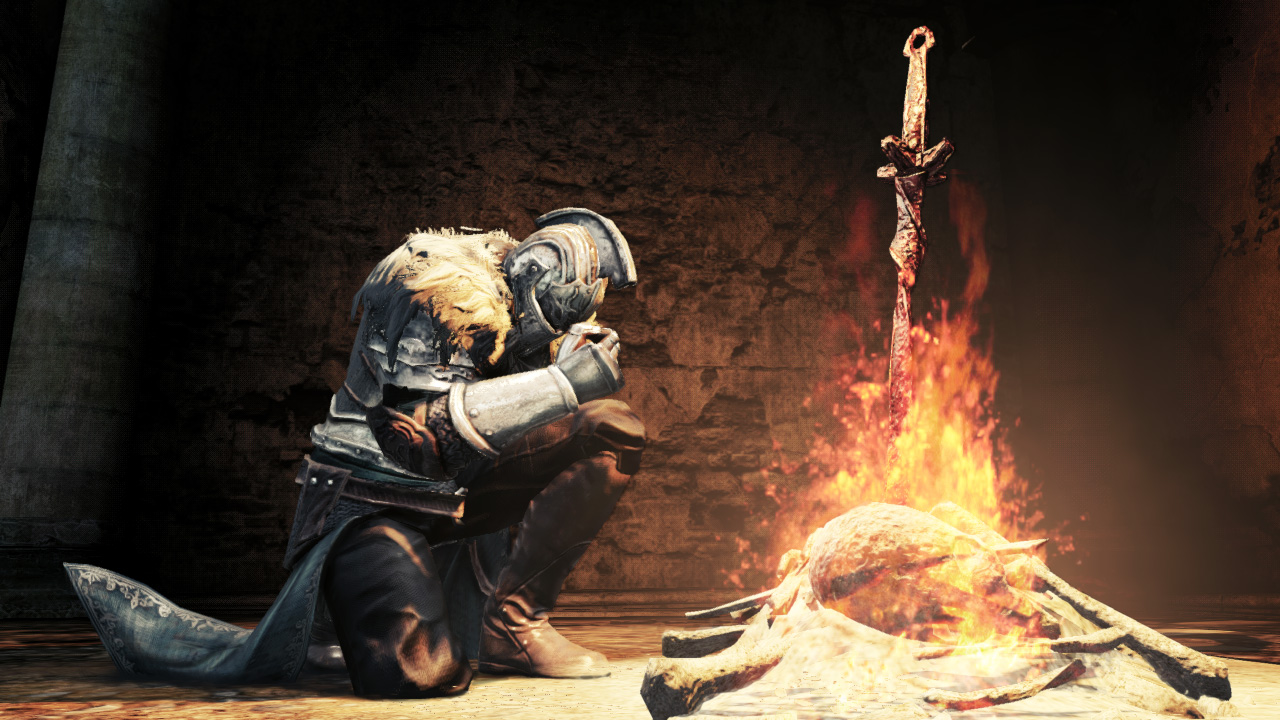# Advent Of Code - Day 7#

## Day 7 - Part 1#

Today we got a bit of respite with an easy one. Sort of like a Dark Souls bonfire.Let’s list our constraints.

Today, we face a giant whale and some crabs in submarines which are less than effective. If they only move horizontally I have no idea how they’ve gotten as far as you. Maybe they pivot from time to time. Or they are indigenous to this region only.

In any case, the crab want to rescue you by blasting a giant hole into the ground in which you can escape. For that they need to align to a position. We’ll have to try each position for the crabs to align to to find the one which requires the least amount of fuel ( steps ) to get to from each of the crabs.

• least amount of fuel
• from each position of the crabs

Okay, this isn’t so bad. This is a simple `min` search from `a` to `b`. Which is basically the lowest crab position to the highest crab position. We’ll try each one of them and which ever produced the least steps for each crab to take, wins.

Let’s see some code. First, we parse the input. Just a `strings.Split(line, ",")` this time. Then, we get the min and the max of the values to look for. This is simply a `for ... if v < min ; if v > max`. Nothing serious.

Then we get to the meat. From the minimum, to the maximum, we calculate how much it would take to move to that position. The fuel is basically, the distance taken from a to b as an absolute value. `abs(a-b)`. This is how that looks like:

``````	for i := min; i <= max; i++ {
currFuel := 0
for _, crab := range crabs {
v := abs(i - crab)
currFuel += v
}
if currFuel < minFuel {
minFuel = currFuel
}
}
``````

We add all that together as our fuel and compare it to the smallest we found so far. And that’s it. We have the solution for part1. Bring it on part 2!

## Day 7 - Part 2#

Okay, now we get to the fun stuff. Turns out, we actually have no idea how crab submarines work. Surprise, surprise. But they tell us that for each move they use +1 fuel. So 1 or the first, 2 for the second, 3 for the third, etc. We do some quick thinking and find that basically, each move requires +1 compared to the previous move. Now… You might be tempted to change around your looping. But remember. Look for patterns! 1, 2, 3, 4…. this is a base number sequence. And what you need is add 1, then 2, then 3, then 4 to the final sum for each move. So basically just sum the number sequence and add it to the end result.

Here, the novice might be tempted to add the numbers with a for loop. But, if you remember your math class, there is a formula for that. It’s called the Gauss formula. But that’s just a fancy pants words for a base number sequence sum. It’s (n*(1+n))/2. Or better, (n*(a1+a2))/2. You can look it up further here.

With that, we simply create a small function value to calculate this:

``````	sum := func(n int) int {
return (n * (1 + n)) / 2
}
``````

And we change our loop a tiny bit:

``````	for i := min; i <= max; i++ {
currFuel := 0
for _, crab := range crabs {
v := sum(abs(i - crab)) // change this to add sum
currFuel += v
}
if currFuel < minFuel {
minFuel = currFuel
}
}
``````

And that’s it! With that, we have our number. Submit to AOC and that’s another star in the bag!

The repository for all my solutions for AOC 2021 can be found here.